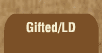Mathematics and Dyslexia

Tips for Learning the Times Tables

Tools for the Times Tables & Workbook

Home > Math > times tables practice > Sixes (big - scrambled)

## The Six Times Tables - second set

The digits of the numbers that you can divide by three always add up to a number three will go into. Even numbers always end in 2, 4, 6, 8, or 0. If both of those are true, then 6 will go into the number.

1. 10 x 6=
2. 6 x 6=
3. 6 x 7=
4. 9 x 6=
5. 6 x 8=
6. 7 x 6=
7. 6 x 9=
8. 6 x 6=
9. 8 x 6=
10. 6 x 7=
11. 9 x 6=
12. 6 x 6=
13. 6 x 8=
14. 7 x 6=
15. 8 x 6=